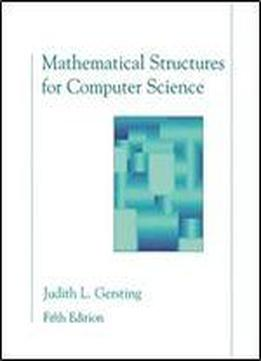# Mathematical Structures For Computer Science: A Modern Treatment Of Discrete Mathematics by Judith L. Gersting / 2002 / English / DjVu

Formal Logic - Proofs, Recursion, and Analysis of Algorithms - Sets, Combinatorics, and Probability - Relations, Functions, and Matrices - Graphs and Trees - Graph Algorithms - Boolean Algebra and Computer Logic - Modeling Arithmetic, Computation, and Languages - Appendix A Summation Notation - Appendix B The Logarithm Function - Answers to Practice Problems - Answers to Selected Exercises - Answers to Self Tests - Index

views: 196